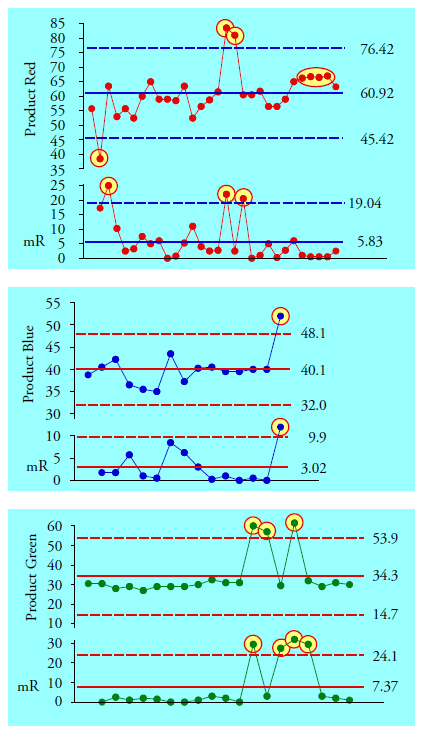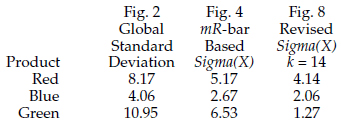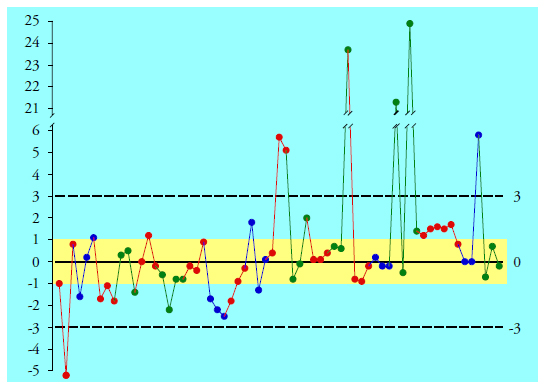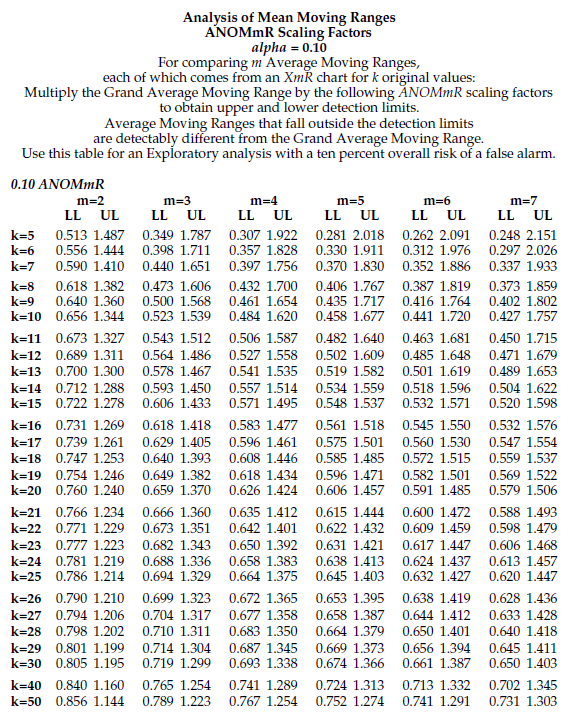#Quality Digest

Featured Product
This Week in Quality Digest Live
Statistics Features
Donald J. Wheeler
What do predictable processes have in common with chaos?
William A. Levinson
People can draw the wrong conclusions due to survivor, survey, and bad news bias.
Donald J. Wheeler
Does your approach do what you need?
Paul Laughlin
Correlation vs. causality
Donald J. Wheeler
In spite of what everyone says to the contrary
Statistics News
New capability delivers deeper productivity insights to help manufacturers meet labor challenges
Day and a half workshop to learn, retain, and transfer GD&T knowledge across an organization
Elsmar Cove is a leading forum for quality and standards compliance
InfinityQS’ quality solutions have helped cold food and beverage manufacturers around the world optimize quality and safety
User friendly graphical user interface makes the R-based statistical engine easily accessible to anyone
Collect measurements, visual defect information, simple Go/No-Go situations from any online device
Good quality is adding an average of 11 percent to organizations’ revenue growth
Ability to subscribe with single-user minimum, floating license, and no long-term commitment
A guide for practitioners and managersBio

Statistics

## Short Run SPC, Part 3

### The robustness of process behavior charts

Published: Monday, February 3, 2020 - 13:03

All articles in this series:

Short Run SPC, Part 1 and Part 2 showed how to use zed charts and difference charts to track the underlying process while making different products. This part will illustrate both the robustness of the zed chart and an incorrect way of standardizing the data from the different products.

### The robustness of process behavior charts

In this section we shall revisit the zed chart from the plant in Europe that was featured in Part One. We do this because, unlike the other examples in parts one and two, this process was being operated unpredictably. This unpredictability introduces some additional steps in the use of the ANOMmR chart. In addition, in retelling this story we shall also discover just how robust the process behavior chart is in practice.

Recall that three products labeled Red, Blue, and Green were produced in one production unit. The data are shown in figure 1 in production order.Figure 1: Data from plant in Europe

When the plant personnel tried to create a standardized chart to visualize the process they used the traditional standardization transformation.Each product value had the product average subtracted off, and the differences were divided by the global standard deviation for that product. The average for Product Red is 60.92 and the standard deviation statistic is 8.17. The average for Product Blue is 40.07 and the standard deviation statistic is 4.06. The average for Product Green is 34.31 and the standard deviations statistic is 10.95. Using these values with the traditional standardization transformation above we get the “standardized” chart in figure 2.Figure 2: “Standardized” chart for 65 batches of three products

Figure 2 shows two runs beyond two sigma (points 33 and 34 and points 50 and 52). So while this chart does contain signals of unpredictable operation, it does not begin to show all the signals we find on the product-specific charts in figure 3.Figure 3: XmR charts for Products Red, Blue, and Green

While the X-charts in figure 3 have seven points outside the limits, these seven points all fall inside the limits in figure 2. This happens because the global standard deviation statistic is the wrong measure of dispersion to use with process behavior charts. Whenever the standardization transformation is based on the global standard deviation most signals will be hidden.

To create a standardized process behavior chart we must use the zed transformation rather than the standardization transformation. The zed transformation for individual values uses a measure of dispersion known as Sigma(X) that is based upon either the average moving range or a median moving range:The details were given in Part One of this series, but to recap, the nominal values for Products Red, Blue, and Green are 60, 40, and 30 respectively. The average moving range for Product Red is 5.83, so Sigma(X) is 5.83/1.128 = 5.17 for Red. The average moving range for Product Blue is 3.02, so Sigma(X) is 3.02/1.128 = 2.67 for Blue. The average moving range for Product Green is 7.37, so Sigma(X) is 7.37/1.128 = 6.53 for Green. When these product specific zed transformations are applied to the 65 original values in figure 1 we end up with the zed chart in figure 4.Figure 4: Figure 4: Zed chart for 65 batches of three products

Figure 4 shows the signals found in figure 3: the seven points outside the limits and the run beyond one-sigma for Product Red. In addition it also shows another run beyond one-sigma for Products Blue and Red that is not seen in figure 3. So how we estimate dispersion can have a dramatic effect upon the chart.

Figure 5 compares the global standard deviation values used in creating figure 2 with the Sigma(X) values used for figure 4. Since we divide by these measures of dispersion, the values in figure 2 are deflated relative to those in figure 4 as shown. It is this automatic deflation of the values that causes figure 2 to hide the signals found in figures 3 and 4.Figure 5: Different estimates of dispersion used

While figure 4 does a much better job of revealing the process behavior than does figure 2, figure 4 is not perfect. The range charts in figure 3 show that each of the average moving ranges has been inflated by one or more points above the upper range limits. This means that the Sigma(X) values in figure 5 are also inflated and the zed chart in figure 4 is contaminated.

In spite of this contamination the zed chart is robust enough to separate the potential signals from the probable noise. When we can do this we have an adequate basis for taking action to improve the process. Since the purpose for using a process behavior chart is to know when to take action, figure 4 is good enough. Perfection is not required. As long as we follow the prescribed computations we can get limits that are good enough to identify the potential signals, and we can do this even when we use imperfect data to compute those limits. This robustness, this ability to get “good limits from bad data” is what makes the charts effective and useful for process characterization.

### Estimation vs. characterization

But when it comes to estimating the process variation the contamination described above can be a problem. While the prescribed computations for the zed chart are robust enough for the charts to work as intended, the contamination introduced by the exceptional values can distort our estimates of process parameters. So when comparing variation from one product to another we may need to first decontaminate our statistics.

For Product Red the average moving range is 5.828. But if we delete the four largest moving ranges and the last five small moving ranges (from the run beyond one sigma) the average of the 20 remaining moving ranges is 3.91.

For Product Blue the average moving range was 3.016. But if we delete the one excessively large moving range the average of the 13 remaining moving ranges is 2.325.

For Product Green the average moving range was 7.367. But if we delete the four excessively large moving ranges the average of the 15 remaining moving ranges is 1.421. These revised average moving ranges will provide better estimates of the process variation for the three products than the ones we used in creating the zed chart in figure 4.

Before we create an ANOMmR chart to compare the variation across products we not only need to use decontaminated statistics, but we also need to have the same baseline lengths. Since our decontaminated estimate for Product Blue has only 13 moving ranges, we truncate the other two sets of moving ranges to use only the first 13 values used above for the decontaminated estimates.

For Product Red we use 13 moving ranges between batch 4 and batch 16 to get an average moving range of 4.673. And for Product Green we use the first 13 moving ranges within the limits to get an average of 1.426.

Using these three revised average moving ranges, our grand average moving range becomes 2.808. For m = 3 baselines with k = 14 values each our 10-percent ANOMmR scaling factors from the table below are 0.593 and 1.450. The resulting ANOMmR chart is found in figure 6.Figure 6: ANOMmR chart for the three products

Thus, we find that the different products have detectably different amounts of variation, making the zed chart a necessity for tracking the underlying process. Dividing each of these average moving ranges by 1.128 gives the revised Sigma(X) values in the last column of figure 7. Comparing the first column with the last we find that the global standard deviation statistics are two to eight times larger than the revised estimates of dispersion.Figure 7: Different estimates of dispersion

### Polishing the zed chart

Figure 2 shows a standardization chart done wrong. Figure 4 shows how the use of the zed transformations avoids burying the signals and properly separates the signals of process changes from the routine variation of the production process. But could we polish up the zed chart by using our less contaminated estimates of dispersion developed for the ANOMmR chart? Yes, we could, but we seldom need to do so for the following reason.

Process behavior charts are intended for process characterization rather than estimation. If you have already found signals, then rather than revising the limits, the proper response is to take action to identify the assignable causes and then either control these causes or compensate for them in production. Any additional signals you might find by polishing the limits will always be the smaller signals. If you are already taking care of the larger signals, you will naturally recompute the limits as changes are made to the process, and eventually you will get around to the smaller signals. And if you are not taking care of the larger signals, then you certainly do not need to find the smaller signals. Thus, when the process is characterized as unpredictable, the revision of the limits is moot. Action is required.

On the other hand, when the process is reasonably predictable, your estimates of the parameters will already be as good as the amount of data will allow them to be, and there is no need to revise the limits.

Nevertheless, to further illustrate both the sensitivity of the zed transformation and the insensitivity of the traditional standardization transformation, we will polish the limits for our zed chart from figure 4. Using the target values of 60, 40, and 30 respectively, and the revised Sigma(X) values from figure 7, we get the zed-chart in figure 8.Figure 8: Zed chart using revised estimates of Sigma(X)

Figure 8 shows that this process is subject to excursions of up to 25 sigma on the high side and excursions of more than five sigma on the low side. Any process that meanders around over a range of 30 sigma represents a huge opportunity for improvement since no process needs elbow room in excess of six sigma when it is being operated predictably.

Yet figure 2, reproduced here as figure 9, shows all of these points falling within the three sigma limits. This illustrates how completely the traditional standardization transformation buries signals—a running record that spanned 30 standard deviations was compressed five-fold so that it would fit within a span of six standard deviations!

This is precisely what the global standard deviation is supposed to do. Since it is built on the assumption that the data are completely homogeneous, it will always do its best to shoe-horn every histogram into the interval [Average ± 3 Global Standard Deviations].Figure 9: “Standardized” chart for 65 batches of three products

The traditional standardization transformation is a steam roller that will successfully flatten things out even when the data contain outliers that are up to 25 standard deviations away from the average!

But rather than hiding the signals by sweeping them under the rug, the purpose of a process behavior chart is to examine the data for evidence of a lack of homogeneity. This is why you should never, ever use a global standard deviation statistic with a process behavior chart—it is simply incompatible with the objective of the charts.

The original zed chart in figure 4 is not completely free of the contaminating effects of the many large ranges. However, in spite of this contamination, it correctly identified all of the potential signals. Polishing the zed transformations as we did in figure 8 did not change the story already told by figure 4, but it did show the magnitude of the process changes with greater clarity.

This is why we say the process behavior chart approach is robust. As long as we use the prescribed computations we will get a chart that is good enough to identify potential signals (e.g., figure 4). This will allow us to begin to operate our processes up to their full potential. We don’t have to have the exact limits, and neither do we have to have the exact zed transformations in order to do this. As long as we use the two-point moving ranges to characterize dispersion when working with individual values, we can get good limits from bad data.

### Summary

When seeking to create a standardized chart it is important to avoid the proscribed computations. The traditional standardization transformation taught in introductory classes in statistics is inappropriate when creating a standardized process behavior chart. When working with individual values, the zed transformations will always use estimates of dispersion that are based on two-point moving ranges.

This is the reason standardized process behavior charts are called zed charts. The label “z” is already attached to the traditional standardization transformation, and as we saw in figure 9, this “z-transformation” is completely incorrect for use with any process behavior chart.

So, before you use any software for “standardized charts” you need to make sure that the estimates of dispersion are not based on product-by-product global standard deviation statistics. A good way to discover if the software does standardized charts correctly is to use the data in figure 1 with the software and see whether you end up with figure 2 or figure 4.For additional tables see “Short Run SPC, Part 2,” Quality Digest, Jan. 6, 2020.

### Discuss